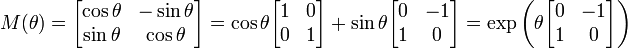# 【Unity】改变向量的方向而不改变其大小/// <summary>
/// 旋转向量，使其方向改变，大小不变
/// </summary>
/// <param name="v">需要旋转的向量</param>
/// <param name="angle">旋转的角度</param>
/// <returns>旋转后的向量</returns>
private Vector2 RotationMatrix(Vector2 v, float angle)
{
var x = v.x;
var y = v.y;
var sin = Math.Sin(Math.PI * angle / 180);
var cos = Math.Cos(Math.PI * angle / 180);
var newX = x * cos + y * sin;
var newY = x * -sin + y * cos;
return new Vector2((float)newX, (float)newY);
}

posted @ 2015-12-05 17:45  妖刀Dreamcast  阅读(...)  评论(... 编辑 收藏